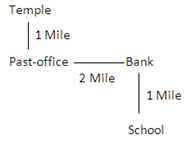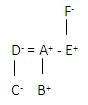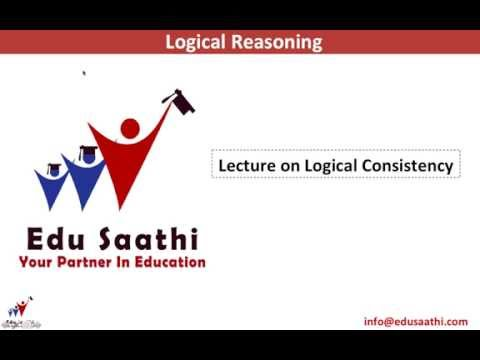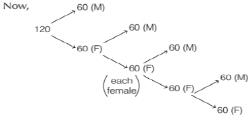Courses

# Practice Test: Logical Reasoning - 2

## 41 Questions MCQ Test Logical Reasoning for CLAT | Practice Test: Logical Reasoning - 2

Description
This mock test of Practice Test: Logical Reasoning - 2 for CLAT helps you for every CLAT entrance exam. This contains 41 Multiple Choice Questions for CLAT Practice Test: Logical Reasoning - 2 (mcq) to study with solutions a complete question bank. The solved questions answers in this Practice Test: Logical Reasoning - 2 quiz give you a good mix of easy questions and tough questions. CLAT students definitely take this Practice Test: Logical Reasoning - 2 exercise for a better result in the exam. You can find other Practice Test: Logical Reasoning - 2 extra questions, long questions & short questions for CLAT on EduRev as well by searching above.
QUESTION: 1

### The age of Ram is twice the age of Shyam and half the age of Sohan, Shyam is elder than Mohan. Who is the eldest?

Solution:

Let the age of Shyam = x year
Then, age of Ram = 2x year
and age of Sohan = 4x year
∴ 4x > 2x > x
On arranging the above data, we get
∴ Sohan > Ram > Shyam > Mohan
So, Sohan is the eldest.

QUESTION: 2

Solution:
QUESTION: 3

### Temple is one mile north from the post office and teh bank is 2 miles east from the post office. If the school is a mile south from the bank, then in what direction is the post office from the school?

Solution:QUESTION: 4

Sunil is twice the age of Swaraj and Naveen is two years younger than Swaraj. If Manoj is one year younger to Sawraj, who is youngest of all?

Solution:

EXP:  Sunil = 2 (Swaraj)

Naveen = Swaraj – 2

Manoj = Swaraj – 1

Let the age of Swaraj = x

Sunil = 2x ; Naveen = 2x – 2

Manoj = 2x – 1

By comparison ; Swaraj is youngest.

QUESTION: 5

In an examination, Soni scored higher than Mona but lower than Pushpa. Pushpa got five marks less than Shobha whereas Shobha and Vibha got equal marks. Who has got the lowest score in examination?

Solution:

EXP: Shobha = Vibha > Pushpa > Sunil > Mon

QUESTION: 6

Shankar’s income is more than that of Shekhar but is equal to that of Santosh. Shekhar’s income is less than that of Suresh but more than that of Raman. If Shankar’s income is less than that of Suresh, then whose income is the highest?

Solution:

EXP: Suresh > Shankar = Santosh > Shekhar > Raman

QUESTION: 7

Ram and Shyam have some rupees. Ram told Shyam that if he gave him one rupee he would have exactly double of what Shyam had. Shyam told Ram that if he (Ram) gave one Rupee to him, both would have an equal amount. How many rupees Ram and Shyam have?

Solution:
QUESTION: 8

A, B, C and D are four wooden poles. If A is longer than C, B is smaller than D, and C longer than D, which is the smallest pole?

Solution:

EXP: A > C > D > B

QUESTION: 9

White is to snow as black is to

Solution:

EXP: As white is the colour of snow so Black is the colour of coal

QUESTION: 10

Eye is to head as window is to

Solution:

EXP: As eye is to the head so window is to wall.

QUESTION: 11

Here are five questions each followed by a set of proposition of which you are to choose the right answer by encircling (O) the right answer.

Q.
Since all rabbits that I have seen have short tails, all rabbits probably have short tails.

Which of the following most closely parallels the kind of reasoning used in the sentence above?

Solution:
QUESTION: 12

Here are five questions each followed by a set of proposition of which you are to choose the right answer by encircling (O) the right answer.

Q.
Because most references to dogs by characters in Shakespeare’s plays are derogatory, it has been argued that Shakespeare did not like dogs. Which of the following is the strongest objection to the argument above.

Solution:
QUESTION: 13

Here are five questions each followed by a set of proposition of which you are to choose the right answer by encircling (O) the right answer.

Q.
If Raman is born in the New York State, then he is a citizen of the United States.

The statement above can be deduced logically from which of the following statements.

Solution:
QUESTION: 14

Here are five questions each followed by a set of proposition of which you are to choose the right answer by encircling (O) the right answer.

Q.
There is no reason to rule out the possibility of life on Uranus. We must then undertake exploration of that planet. The argument above assumes which one of the following

Solution: The given statements already says that they must undertake some measures to explore into space. Thus correct answer is C.Option A fails because there is no reason to disbelieve that life does not exist on Uranus.B says the same thing as A.
QUESTION: 15

Here are five questions each followed by a set of proposition of which you are to choose the right answer by encircling (O) the right answer.

Q.
University officials have tried to suppress the amount of drugs used by students. However, one has to only look at the decline in the success rate of students during the last decade to realize the full extent of the drug problem in campuses.

Which of the following, if true, weakens the conclusion above for decline in success rate of students independent of drug use by students?

Solution:
QUESTION: 16

Here are five questions each followed by a set of proposition of which you are to choose the right answer by encircling (O) the right answer.

Q.
My father is a successful lawyer, but he has become a patient of hypertension over the years.

I have decided not to become a lawyer.

Which of the following is based on similar logic.

Solution:
QUESTION: 17

Here are five questions each followed by a set of proposition of which you are to choose the right answer by encircling (O) the right answer.

Q. The Constitution has given the right to freedom of speech. Some people create communal disharmony while exercising this right.

Solution:
QUESTION: 18

Here are five questions each followed by a set of proposition of which you are to choose the right answer by encircling (O) the right answer.

Q.
Prices have gone up despite the cut in excise duties.

Which of the following has the similar logic?

Solution:
QUESTION: 19

Here are five questions each followed by a set of proposition of which you are to choose the right answer by encircling (O) the right answer.

Q.
“Idle brain is a devil’s workshop”. Which of the following has the similar logic?

Solution:
QUESTION: 20

Here are five questions each followed by a set of proposition of which you are to choose the right answer by encircling (O) the right answer.

Q.
“Smoking is injurious to health; still people smoke”. Which of the following has a similar logic?

Solution:
QUESTION: 21

Fifty books belonging to different subjects, viz., History (8), Geography (7), Literature (13), Psychology (8) and Science (14), are placed on a shelf. They are arranged in an alphabetical order subject to the condition that no two books of the same subject are placed together to so long as books of other subjects are available. Unless otherwise mentioned, all counting is done from the left.​

Q.
Which subjects does the 40th book belong to?

Solution:
QUESTION: 22

Fifty books belonging to different subjects, viz., History (8), Geography (7), Literature (13), Psychology (8) and Science (14), are placed on a shelf. They are arranged in an alphabetical order subject to the condition that no two books of the same subject are placed together to so long as books of other subjects are available. Unless otherwise mentioned, all counting is done from the left.​

Q. What is the position of the last book in Psychology?

Solution:

The correct option is A.

the 12 th book from left should be the 39 th book from right so answer is psychology

QUESTION: 23

Fifty books belonging to different subjects, viz., History (8), Geography (7), Literature (13), Psychology (8) and Science (14), are placed on a shelf. They are arranged in an alphabetical order subject to the condition that no two books of the same subject are placed together to so long as books of other subjects are available. Unless otherwise mentioned, all counting is done from the left.​

Q.
Counting from the right to which subject does the 39th book belong?

Solution:

Because Geography books are only 7 and the series of geography book ends by 35 books only . Then comes history, literature , psychology,science as 36,37,38,39 and following that series the 40 th book must be literature .

QUESTION: 24

For each of the following questions, there are two words and four alternative answers. In each of the alternative answers there are two words. Find out the alternative whose two words have the same relation as in the two words given in the beginning,

Q.
Crime: Punishment

Solution:
QUESTION: 25

For each of the following questions, there are two words and four alternative answers. In each of the alternative answers there are two words. Find out the alternative whose two words have the same relation as in the two words given in the beginning,

Q.
Mistake: Void

Solution:
QUESTION: 26

For each of the following questions, there are two words and four alternative answers. In each of the alternative answers there are two words. Find out the alternative whose two words have the same relation as in the two words given in the beginning,

Q.
Freedom: Slavery

Solution:

The correct option is A.
Safety is opposite of Trouble and Slavery is opposite of Freedom.

QUESTION: 27

For each of the following questions, there are two words and four alternative answers. In each of the alternative answers there are two words. Find out the alternative whose two words have the same relation as in the two words given in the beginning,

Q.
Appellant: Respondent

Solution:
QUESTION: 28

In each of the following questions, there are two words and four alternative answers. In each alternative answer there are two words. Find out the alternative wherein two words have the same relation as in the two words given in beginning.

Q.
Criminal law: Punishment

Solution:

The correct option is C.
This is the cause and effect relationship.
Rights Restoration is the process of restoring voting rights to persons with prior felony convictions who lost their rights under felony disenfranchisement. It may also refer to additional civil rights that are taken away upon conviction, such as holding public office and serving on a jury.

QUESTION: 29

In each of the following questions, there are two words and four alternative answers. In each alternative answer there are two words. Find out the alternative wherein two words have the same relation as in the two words given in beginning.

Q.
President: Center

Solution:
QUESTION: 30

In each of the following questions, there are two words and four alternative answers. In each alternative answer there are two words. Find out the alternative wherein two words have the same relation as in the two words given in beginning.

Q.
Mens rea: Actus Reus

Solution:

The correct option is B.
Basically, 'mens rea' refers to the mindset being the 'intention' and 'actus reus' refers to the crime being the 'act' hence by comparing the subject to the analysis.

QUESTION: 31

In each of the following questions, there are two words and four alternative answers. In each alternative answer there are two words. Find out the alternative wherein two words have the same relation as in the two words given in beginning.

Q.
In a certain code language ‘pik da pa’ means ‘where are you’; da na fa’ means ‘you may cme’ ‘na ka sa’ means ‘he may go’, which of the following means ‘come’ in that code language?

Solution:
QUESTION: 32

Flower is to Bouquet as Minister is to ________

Solution:
QUESTION: 33

In the following series which is the 5th letter to the left of 14th letter from your right?
ABCDEFGHIJKLMNOPQRSTUVWXYZ.

Solution:
QUESTION: 34

Three men (Tej, Partha, and Jeeva) and three women (Eleena, Anita and Kavita) are spending a few months at a hillside. They are to stay in a row of nine cottages, each one living in his or her own cottage. There is nobody else staying in the same row of houses.

1) Anita, Tej and Jeeva do not want to stay in any cottage, which is at the end of the row.

2) Anita is unwilling to stay beside any occupied cottage.

3) Kavita is next to Partha and Jeeva.

4) Between Anita and Jeeva’s cottage-there is just one vacant house.

5) None of the girls occupy adjacent cottages.

6) The house occupied by Tej is next to an end cottage.

Q.
Which of the above statements can be said to have been derived from two other statements?

Solution:

The correct option is D.
Arrangement is as follows-
Tej,Parth,kavita, jeeva, vacant,anita, vacant, ellena,vacant

QUESTION: 35

Three men (Tej, Partha, and Jeeva) and three women (Eleena, Anita and Kavita) are spending a few months at a hillside. They are to stay in a row of nine cottages, each one living in his or her own cottage. There is nobody else staying in the same row of houses.

1) Anita, Tej and Jeeva do not want to stay in any cottage, which is at the end of the row.

2) Anita is unwilling to stay beside any occupied cottage.

3) Kavita is next to Partha and Jeeva.

4) Between Anita and Jeeva’s cottage-there is just one vacant house.

5) None of the girls occupy adjacent cottages.

6) The house occupied by Tej is next to an end cottage.

Q.
How many of them occupy cottages next to a vacant cottage?

Solution:
QUESTION: 36

A, B, C, D, E and F is a group of six persons in a family. Number of gents is same as the number of ladies.

A & E are sons of F.

D is the mother of two children of whom, one is a boy and the other is a girl.

B is the son of A.

There is only one pair of married people in the group.

Q.
Which of the following groups consists of ladies?

Solution:QUESTION: 37

Three men (Tej, Partha, and Jeeva) and three women (Eleena, Anita and Kavita) are spending a few months at a hillside. They are to stay in a row of nine cottages, each one living in his or her own cottage. There is nobody else staying in the same row of houses.

1) Anita, Tej and Jeeva do not want to stay in any cottage, which is at the end of the row.

2) Anita is unwilling to stay beside any occupied cottage.

3) Kavita is next to Partha and Jeeva.

4) Between Anita and Jeeva’s cottage-there is just one vacant house.

5) None of the girls occupy adjacent cottages.

6) The house occupied by Tej is next to an end cottage.

Q.
How many of them occupy cottages next to a vacant cottage?

Solution:

B is the correct option. According to the question - Jeeva ..Anita..tej..eleena are all occupying cottages next to vacant one..
acc to the question..the respected positions are..
1. Kavita 2.partha 3.jeeva 4. Vacant  5.anita 6. Vacant 7.tej 8. Vacant 9. eleena

QUESTION: 38

A, B, C, D, E and F is a group of six persons in a family. Number of gents is same as the number of ladies.

A & E are sons of F.

D is the mother of two children of whom, one is a boy and the other is a girl.

B is the son of A.

There is only one pair of married people in the group.

Q.
​Who is the uncle of C?

Solution:QUESTION: 39

A, B, C, D, E and F is a group of six persons in a family. Number of gents is same as the number of ladies.

A & E are sons of F.

D is the mother of two children of whom, one is a boy and the other is a girl.

B is the son of A.

There is only one pair of married people in the group.

Q.
Who is the husband of D?

Solution:QUESTION: 40

A, B, C, D, E and F is a group of six persons in a family. Number of gents is same as the number of ladies.

A & E are sons of F.

D is the mother of two children of whom, one is a boy and the other is a girl.

B is the son of A.

There is only one pair of married people in the group.

Q.
Which of the following are the pair of children of D?

Solution:QUESTION: 41

A, B, C, D, E and F is a group of six persons in a family. Number of gents is same as the number of ladies.

A & E are sons of F.

D is the mother of two children of whom, one is a boy and the other is a girl.

B is the son of A.

There is only one pair of married people in the group.

Q.
Who is the granddaughter of F?

Solution:Track your progress, build streaks, highlight & save important lessons and more!

### Similar Content### Related tests